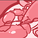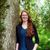# Connectivity & Data Prep

Discussion board where members can learn more about Qlik Sense Data Connectivity.

Announcements
Our May 2021 end-to-end product release from Data Integration to Data Analytics is out! READ DETAILS
cancel
Showing results for
Search instead for
Did you mean:Creator III

## Error with "For i = 0 to (vNumberOfRows - 1)"

Hello!

I have the following script:

[Variables-Formulas]:
LOAD
[Variable-Formula],
Expression,
Usage
FROM [lib://Matrix Variables-Formulas (uhealth_54171224)/Matrix Variables-Formulas.xlsx]
(ooxml, embedded labels, table is [Variables-Formulas]);
LET vNumberOfRows = NoOfRows('Variables-Formulas');
For i = 0 to (vNumberOfRows - 1)
LET vVariable_Name = Peek('Variable-Formula',i,'Expression');
LET [\$(vVariable_Name)] = Peek('Expression',i,'Expression');
Next

NullAsValue "UMHC SCCC",
"UHealth Tower",
"UMHC BPEI",
"UMHC LFMC",
"UMHC Radiology",
"UMHC Applebaum",
"UMHC Ear Institute",
"UMHC Pain Clinic",
"UMHC Safie",
"UMHC IR Clinic",
"UMHC Coral Spring",
"UMHC Deerfield",
"UMHC Hollywood",
"UMHC Kendall",
"UMHC Plantation",
"UMHC Marlin Park";
SET NullValue = '0';

[Map EC-LS-EM]:
MAPPING Load *
Inline [
EC-LS-EM, Description
EC, Environment of Care
LS, Life Safety
EM, Emergency Management
];

Which had been working fine but now I am getting this error:

The following error occurred:

The error occurred here:
For i = to

I am not really sure what is happening.

Any ideas?

THANK YOU!

Labels (2)

• ### Qlik Sense

12 RepliesMVP

You need to use dollar sign expansion around the variable name:

For i = 0 to (\$(vNumberOfRows) - 1)Creator III
Author

Hi,

When I do that, I get this error:

The following error occurred:
Unexpected token: ')', expected one of: '(', 'ZTestw_z', 'OPERATOR_PLUS', 'OPERATOR_MINUS', 'not', 'bitnot', 'LITERAL_NUMBER', ...

The error occurred here:
For i = 0 to (>>>>>>)<<<<<<MVP

That error is coming from the values you are trying to store in vVariable_Name and [\$(vVariable_Name)].  Change those from LET to SET.

(And make sure to leave the dollar sign expansion on the FOR.)Creator III
Author

Ok So I changed them to SET:

For i = 0 to (\$(vNumberOfRows - 1))
SET vVariable_Name = Peek('Variable-Formula',i,'Expression');
SET [\$(vVariable_Name)] = Peek('Expression',i,'Expression');
Next

And I still get that error?  😥MVP

You have some incorrect arguments in your PEEK functions.  The third argument should be table name:

[Variables-Formulas]:
LOAD
[Variable-Formula],
Expression,
Usage
FROM [lib://Matrix Variables-Formulas (uhealth_54171224)/Matrix Variables-Formulas.xlsx]
(ooxml, embedded labels, table is [Variables-Formulas]);
LET vNumberOfRows = NoOfRows('Variables-Formulas');
For i = 0 to (\$(vNumberOfRows) - 1)
LET vVariable_Name = Peek('Variable-Formula',i,'Variables-Formulas');
LET [\$(vVariable_Name)] = Peek('Expression',i,'Variables-Formulas');
Next

And leave them as LET statements--the PEEKs won't evaluate otherwise.  I missed that previously.Creator III
Author

Hi thank you, but that is still causing me an error:

The following error occurred:
Unexpected token: ')', expected one of: '(', 'ZTestw_z', 'OPERATOR_PLUS', 'OPERATOR_MINUS', 'not', 'bitnot', 'LITERAL_NUMBER', ...

The error occurred here:
For i = 0 to (>>>>>>)<<<<<<MVP

This example works:

[Variables-Formulas]:
LOAD [Variable-Formula],
[Expression],
[Usage]
INLINE [
Variable-Formula, Expression, Usage
Test 1, min(Usage), 10
Test 2, max(Usage), 20
];

LET vNumberOfRows = NoOfRows('Variables-Formulas');
For i = 0 to (\$(vNumberOfRows) - 1)
LET vVariable_Name = Peek('Variable-Formula',i,'Variables-Formulas');
LET [\$(vVariable_Name)] = Peek('Expression',i,'Variables-Formulas');
Next

SET vNumberOfRows =;
SET i =;
SET vVariable_Name =;

Can you share the Excel file?  It has to do with the values in there that you're trying to pass into the variables.Creator III
Author

Yes of course, I have attached it here.

It is just strange since I haven't changed anything on that sheet.

Thank you for all your help!MVP

This code works fine for me when I run it (no errors and it creates all variables):

``````[Variables-Formulas]:
LOAD [Variable-Formula],
[Expression],
[Usage]
FROM [lib://Desktop/Matrix Variables-Formulas.xlsx]
(ooxml, embedded labels, table is [Variables-Formulas]);

LET vNumberOfRows = NoOfRows('Variables-Formulas');
For i = 0 to (\$(vNumberOfRows) - 1)
LET vVariable_Name = Peek('Variable-Formula',i,'Variables-Formulas');
LET [\$(vVariable_Name)] = Peek('Expression',i,'Variables-Formulas');
Next

SET vNumberOfRows =;
SET i =;
SET vVariable_Name =;``````

What version are you running?  I'm using Desktop April 2019.